SYLLABUS  Previous: 1.3.4 Wave-breaking  Up: 1.3 Prototype problems  Next: 1.4 Numerical discretization

1.3.5 Schrödinger

Slide : [ Schrödinger || VIDEO login]

Choosing units where Planck's constant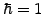and the mass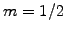, the time-dependent Schrödinger equation clearly appears as a special type of wave / diffusion equation: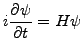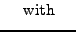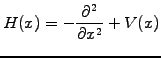(1.3.5#eq.1)

In quantum mechanics, the Schrödinger equation is used to evolve the complex wave-function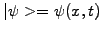to describe the probability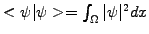of finding a particle in a given interval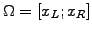. Take the simplest example of a free particle modeled with a wave-packet in a periodic domain and assume a constant potential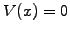. The JBONE applet below shows the evolution of a low energy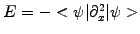(long wavelength) particle that is initially known with a rather good accuracy in space (narrow Gaussian envelope):

the wave-function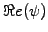(blue line) starts to oscillate, the probability density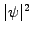spreads out (black line) and the position increasingly becomes uncertain... reproducing the famous uncertainty principle.

 Numerical experiments: Heisenberg's uncertainty principle Reduce the ICWavelength down to 2 and relate an increase in the kinetic energy or the velocity of the wave-packet with a more accurate localization. Reload the applet with a lower kinetic energy ICWavelength=6 and reduce the accuracy of the initial position ICWidth=12 by spreading out the envelope: by spreading out the wave-function, you in effect reduced the uncertainty in the velocity.

SYLLABUS  Previous: 1.3.4 Wave-breaking  Up: 1.3 Prototype problems  Next: 1.4 Numerical discretization